Home MonkeyNotes Printable Notes Digital Library Study Guides Study Smart Parents Tips College Planning Test Prep Fun Zone Help / FAQ How to Cite New Title Request

 Example      Solve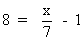Solution :     Rewriting,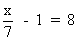Adding 1 to each side,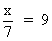Multiplying by 7 to each side, x = 63 Example      Solve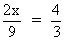Solution :      Multiplying by 9 to both sides,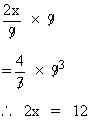Dividing each sides by 2, x = 6 Transposition : Any term of an equation may be moved from one side to the other if the sign proceeding it is changed from + to - or from - to + as the case may be. This movement is called transposition. Example Solve 3 (2x + 1) + 4 = 8x + 1 Solution : Removing brackets, 6x + 3 + 4 = 8x + 1 \ 6x + 7 = 8x + 1 Moving 6x tothe right and 1 to the left side, 7 - 1 = 8x - 6x 6 = 2x i.e. 2x = 6 x = 3 Check : - When x = 3, 3 (2 x + 1) + 4 = 3 (6+1) + 4 = 25 also  8 x +1 = 8 (3) +1 = 25 Index 7.1 Definition7.2 Simultaneous Equations7.3 Inequations (Inequalities)7.4 Absolute Values Chapter 8
 Search: All Products Books Popular Music Classical Music Video DVD Toys & Games Electronics Software Tools & Hardware Outdoor Living Kitchen & Housewares Camera & Photo Cell Phones Keywords: